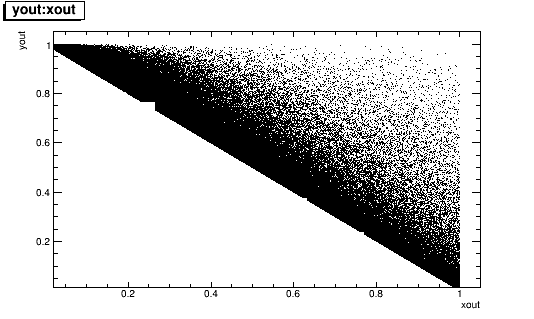# Roofit sampling errors

Dear experts,
I create a C function, and a corresponding TF2 from it. I then bind a pdf to this function, and sample from it. But the sampled data often has large chunks removed from it. Also, when I write this data set to file I find that the values sampled often exceed the limits I define. Lastly, I get numerical integration warnings:
[#0] WARNING:NumericIntegration – RooAdaptiveIntegratorND::dtor(fa1) WARNING: Number of suppressed warningings about integral evaluations where target precision was not reached is 1

A stripped down version of my code is below:

``````const double r = 1.0e-4;
double func(double* xPtr, double par[]){
double x=xPtr;
double y=xPtr;
double val=0;
double term1=0, term2=0;

term1 = 1-y+r*r;
term1 /= x*x;
term1 /= x+y-1-r*r;
term2 =  x*x+2*(1-x)*(1-r*r);
term2 -= 2*x*r*r*(1-r*r)/(x+y-1-r*r);

val = term1*term2;
if(x>.02)val=10e-12;
if(val<0||val>10e4)val=10e-12;
return val;
}

int main(){
genModel();
return 1;
}

void genModel(){
RooRealVar y("y", "y", 2.0*r, 1.0-r*r);
RooRealVar x("x", "x", .02, 1.0);
RooFormulaVar xlo("xlo","1.0-0.5*y-.5*sqrt(y*y-4.0*1.34e-5)",y);
RooFormulaVar xhi("xhi","1.0-0.5*y+.5*sqrt(y*y-4.0*1.34e-5)",y);
x.setRange("triangle",xlo,xhi);

TF2 *fa1 = new TF2("fa1",func,0,1,0,1,2);
RooAbsPdf *funcpdf = bindPdf(fa1,x,y);
RooDataSet *sampled_data=funcpdf->generate(RooArgSet(x,y),1000000);

TFile *outFile = new TFile("sample.root","RECREATE");
TTree *outtree = new TTree("outtree","outtree") ;
Double_t xout = 0, yout = 0;
outtree->Branch("xout",&xout,"xout/D") ;
outtree->Branch("yout",&yout,"yout/D") ;

for(int I=0;I<sampled_data->numEntries();I++) {
const RooArgSet* arg_set = sampled_data->get(I);
xout = arg_set->getRealValue("x");
yout = arg_set->getRealValue("y");
if (xout <(1.0-0.5*yout-.5*sqrt(yout*yout-4.0*1.34e-5))) cout << "x is too low: " << xout << endl;
if (xout >(1.0-0.5*yout+.5*sqrt(yout*yout-4.0*1.34e-5))) cout << "x is too high: " << xout << endl;
outtree->Fill();
}
``````

Now I have created my own class and imported it into RooFit, along with dictionary generation via CINT and so forth. Perhaps this will be better able to handle the integration and range setting.

But do you have any advice on how to fix these problems?

1. Have I implemented the range-setting incorrectly?
2. Should I be worried about the warnings w.r.t. integration, and is there a simple way of doing better?
3. The chunks that are removed from the data are clearly squares, perhaps coming from an underlying foam element that cannot complete. I am looking at the tutorial root.cern.ch/root/html/tutorial … fig.C.html
but if anything trivial jumps out to you that I can do to improve performance/stability, please offer it.

Many Thanks!

I have resolved the range errors through the use of TF1::RejectPoint() in my formulas.

But I have confirmed that the errors in integration are coming from the underlying TFoam–the creation of the pdf and its normalization are not problematic.

Are there any knobs that I can turn on my sampling method that may be helpful? I attach the picture of the sampled data for convenience.

``````double func(double* xPtr, double par[]){
double x=xPtr;
double y=xPtr;
double val=0;
double term1=0, term2=0;

term1 = 1-y+r*r;
term1 /= x*x;
term1 /= x+y-1-r*r;
term2 =  x*x+2*(1-x)*(1-r*r);
term2 -= 2*x*r*r*(1-r*r)/(x+y-1-r*r);

val = term1*term2;
if(x<.02 ||
x>(1-r*r) ||
y<4*r ||
(y>1-r*r))
{TF1::RejectPoint(); return 0;}
if(x<(1.0-0.5*y-.5*sqrt(y*y-4.0*r*r)) ||
x>(1.0-0.5*y+.5*sqrt(y*y-4.0*r*r)))
{TF1::RejectPoint(); return 0;}
if(val<0 || val>10e4)
{TF1::RejectPoint(); return 0;}
return val ;
}
``````

``````  RooRealVar y("y", "y", 2.0*r, 1.0-r*r);